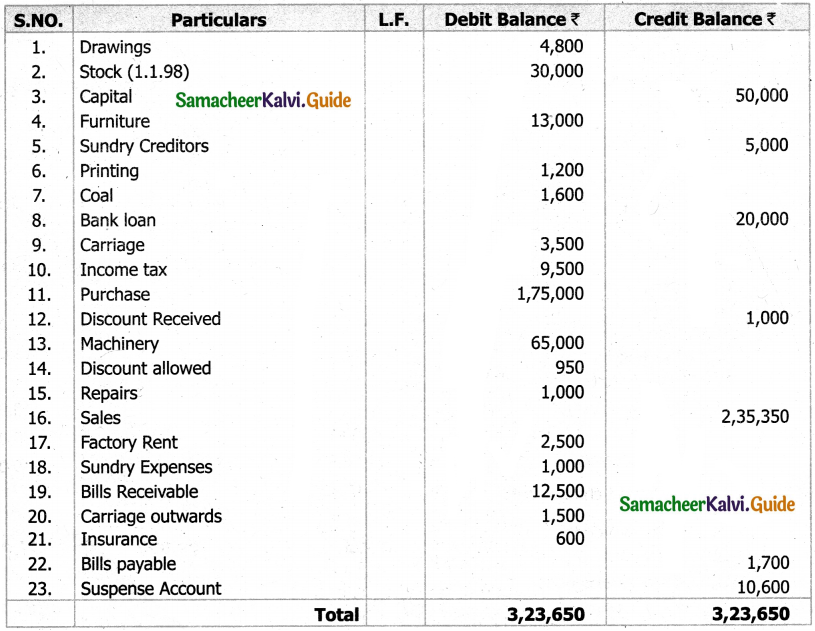Tamilnadu State Board New Syllabus Samacheer Kalvi 11th Accountancy Guide Pdf Chapter 5 Trial Balance Text Book Back Questions and Answers, Notes.

## Tamilnadu Samacheer Kalvi 11th Accountancy Solutions Chapter 5 Trial Balance

### 11th Accountancy Guide Trial Balance Text Book Back Questions and AnswersI. Multiple Choice Questions

Question 1.
Trial balance is a _______.
a) Statement
b) Account
c) Ledger
d) Journal
a) Statement

Question 2.
After the preparation of ledger, the next step is the preparation of _______.
b) Trial balance
c) Journal
d) Profit and loss account
b) Trial balance

Question 3.
The trial balance contains the balances of _______.
a) Only personal accounts
b) Only real accounts
c) Only nominal accounts
d) All accounts
d) All accounts

Question 4.
Which of the following is/are the objective(s) of preparing trial balance?
a) Serving as the summary of all the ledger accounts
b) Helping in the preparation of final accounts
c) Examining arithmetical accuracy of accounts
d) a, b and c
d) a, b and cQuestion 5.
While preparing the trial balance, the accountant finds that the total of the1 credit column is short by Rs. 200. This difference will be _______.
a) Debited to suspense account
b) Credited to suspense account
c) Adjusted to any of the debit balance
d) Adjusted to any of the credit balance
b) Credited to suspense account

Question 6.
A list which contains balances of accounts to know whether the debit and credit balances are matched is _______.
a) Journal
b) Day book
c) Trial balance
d) Balance sheet
c) Trial balance

Question 7.
Which of the following method(s) can be used for preparing trial balance?
a) Balance method
b) Total method
c) Total and Balance method
d) a, b and c
d) a, b and c

Question 8.
The account which has a debit balance and is shown in the debit column of the trial balance is _______.
a) Sundry creditors account
b) Bills payable account
c) Drawings account
d) Capital account
c) Drawings account

Question 9.
The difference of totals of both debit and credit side of trial balance is transferred to:
b) Difference account
c) Suspense account
d) Miscellaneous account
c) Suspense accountQuestion 10.
Trial balance is prepared:
a) At the end of the year
b) On a particular date
c) For a year
d) None of the above
b) On a particular date

II. Very Short Answer Type Questions

Question 1.
What is trial balance?
“A trial balance is a statement, prepared with the debit and credit balances of the ledger accounts to test the arithmetical accuracy of the books”.

Question 2.
Give the format of trial balance.
Trial balance is prepared in the following format under the balance method: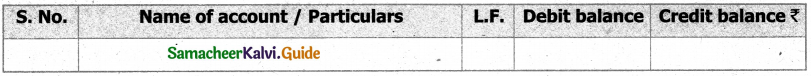Question 3.
What are the methods of preparation of trial balance?
Trial Balance is prepared in the following methods:
Balance method : In this method, the balance of every ledger account either debit or credit, as the case may be, is recorded in the trial balance against the respective accounts. The balance method is widely used, as it helps in the preparation of financial statements.

Total method : Under this method, the total amounts on the debit side of the ledger accounts and the total amounts on the credit side of the ledger accounts are ascertained and recorded in the trial balance. This method is not commonly used as it cannot help in the preparation of financial statements.

Total and Balance method : This method is a combination of both total method and balance method. Under this method, four columns are provided, namely,

• Totals of debit side of the ledger accounts,
• Totals of the credit side of the ledger accounts
• Debit balances of ledger accounts and
• Credit balances of the ledger accounts. This method is not in practice.Question 4.
State whether the balance of the following accounts should be placed in the debit or the credit column of the trial balance:
(i) Carriage outwards
(ii) Carriage inwards
(iii) Sales
(iv) Purchases
(vi) Interest paid
(ix) Capital
(x) Drawings
(xi) Sales returns
(xii) Purchase returns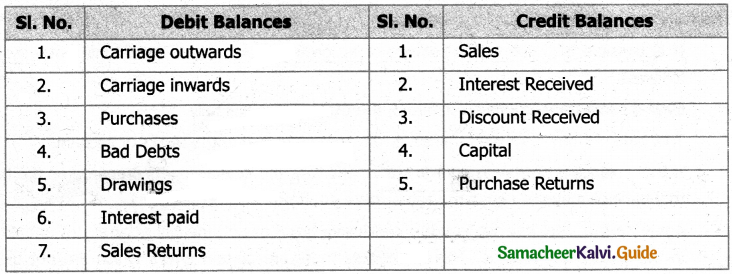Question 1.
What are the objectives of preparing trial balance?
1. Test of arithmetical accuracy:
(i) Trial balance is the means by which the arithmetical accuracy of the book-keeping work is checked.

(ii) When the totals of debit column and credit column in the trial balance are equal, it is assumed that posting from subsidiary books, balancing of ledger accounts, etc. are arithmetically correct.

(iii) There may be some errors which are not disclosed by trial balance.

2. Basis for preparing final accounts : Financial statements, namely, trading and profit and loss account and balance sheet are prepared on the basis of summary of ledger balances obtained from the trial balance.

Location of errors:
(i) When the trial balance does not tally, it is an indication that certain errors have occurred.

(ii) The errors may have occurred at one or more of the stages of accounting process, namely, journalising or recording in subsidiary books, totalling subsidiary books, posting in ledger accounts, balancing the ledger accounts, carrying ledger account balances to the trial balance, totalling the trial balance columns, etc.

(ii) Hence, the errors should be located and rectified before preparing the financial statements.

3. Summarised information of ledger accounts : The summary of ledger accounts is shown in the trial balance. Ledger accounts have to be seen only when details are required in respect of an account.Question 2.
What are the limitations of trial balance?
The following are the limitations of trial balance:

1. It is possible to prepare trial balance of an organisation, only if the double entry system is followed.
2. Even if some transactions are omitted, the trial balance will tally.
3. Trial balance may tally even though errors are committed in the books of account.
4. If trial balance is not prepared in a systematic way, the final accounts prepared on the basis of trial balance may not depict the actual state of affairs of the concern.
5. Agreement of trial balance is not a conclusive proof of arithmetical accuracy of entries made

Question 3.
‘A trial balance is only a prima facie evidence of the arithmetical accuracy of records’. Do you agree with this statement? Give reasons.
1. Yes. Trial Balance helps to check the arithmetical accuracy of the entries made in the accounting records.
In the computerized accounting system, once the transactions are recorded in the journals, all the other records are made simultaneously, i.e. ledger postings, trial balance and final accounts.

2. Hence, arithmetic errors and errors in posting the entries from journal to ledger and further will not occur in computerised accounting.

3. When double entry system is followed, the totals of the debit and the credit columns of the trial balance must be equal. Thus, trial balance helps to check the arithmetical accuracy of entries made in the books of accounts.

IV. Exercises

Question 1.
Prepare a trial balance with the following information: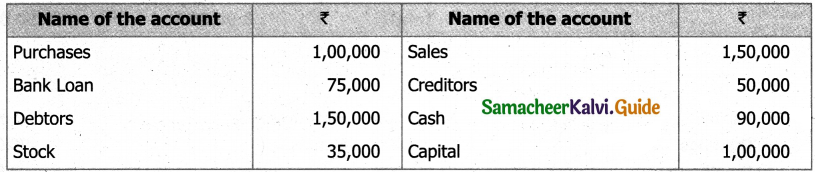Solution:
Trial Balance: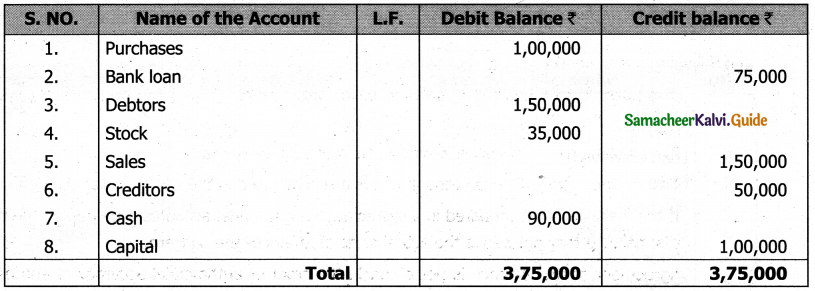Question 2.
Prepare the trial balance from the following information: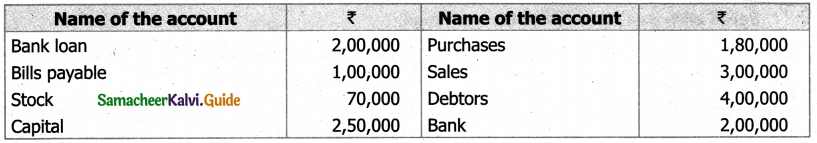Solution:
Trial Balance: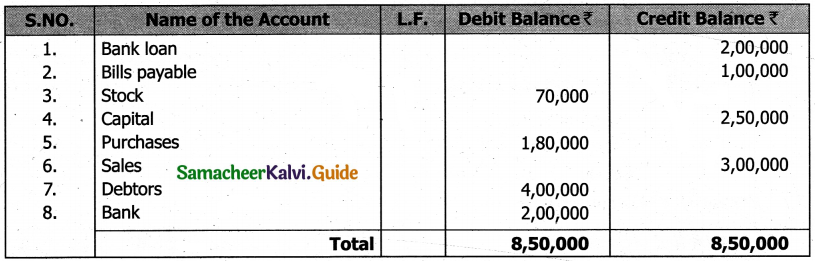Question 3.
Prepare the trial balance from the following balances of Chandramohan as on 31st March, 2017.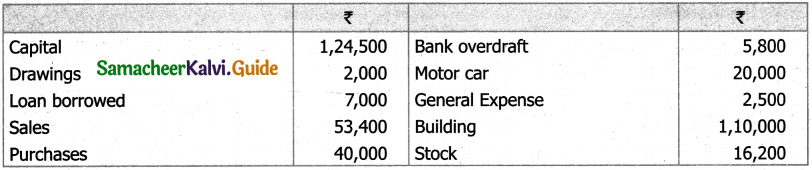Solution:
In the books of Chandramohan Trial balance as on 31st March 2017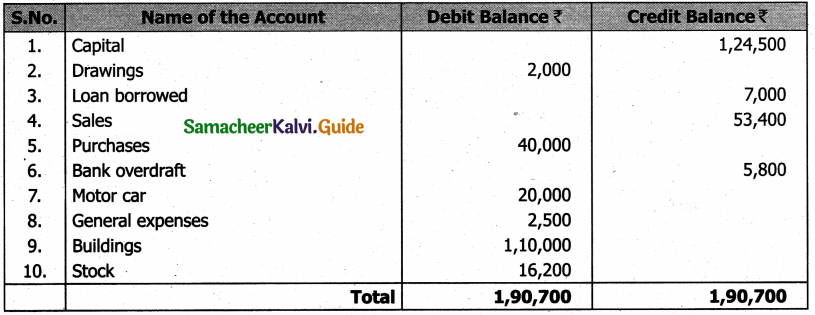Question 4.
Prepare the trial balance from the following balances of Babu as on 31st March, 2016.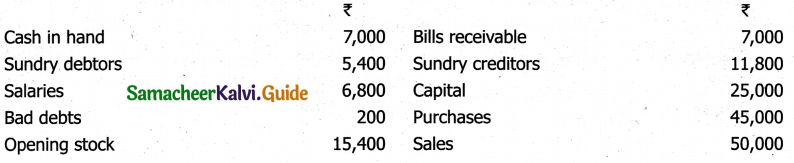Solution:
In The Books of Arjun Trial Balance As On 31st March 2018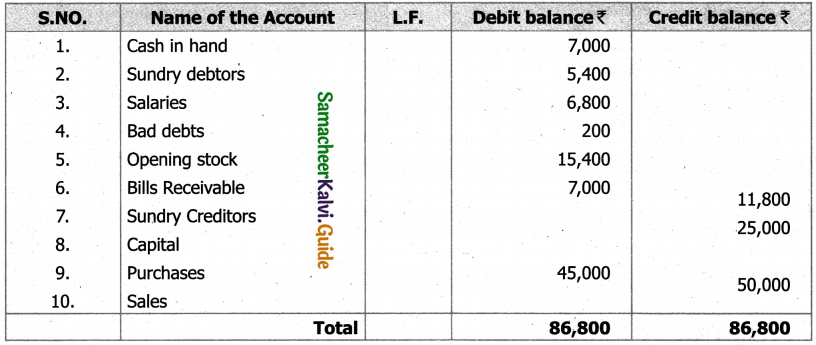Question 5.
From the following balances of Arjun, prepare the trial balance as on 31st March, 2018.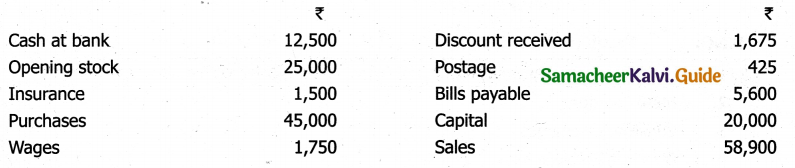Solution:
In The Books of Arjun Trial Balance As On 31st March 2018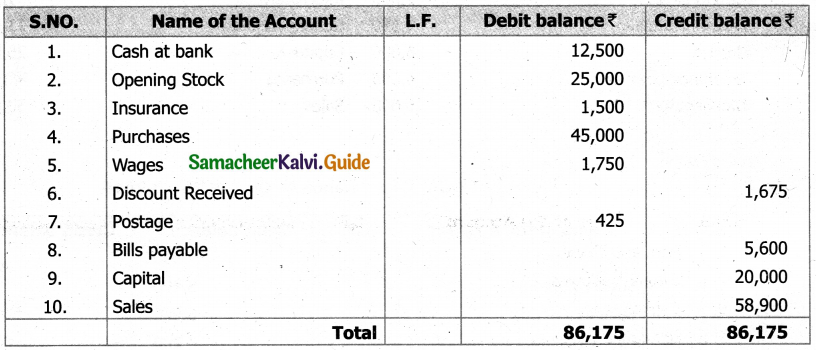Question 6.
Prepare the trial balance from the following balances of Rajesh as on 31st March, 2017.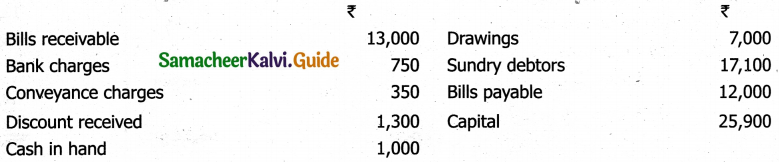Solution:
In The Books of Rajesh Trial Balance as on 31st March 2017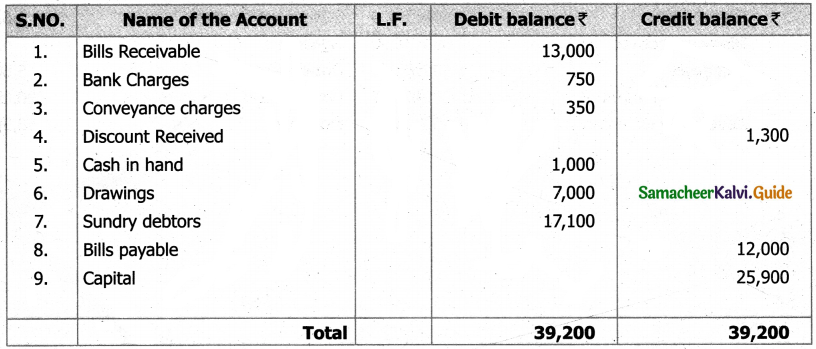Question 7.
Prepare the trial balance from the following balances of Karthik as on 31st March, 2017.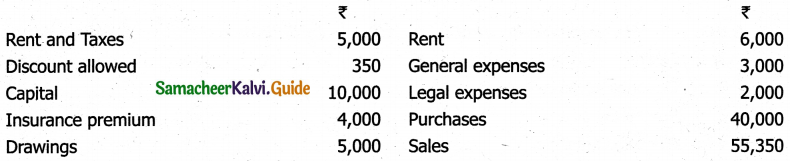Solution:
In the books of Karthik Trial Balance as on 31st March 2018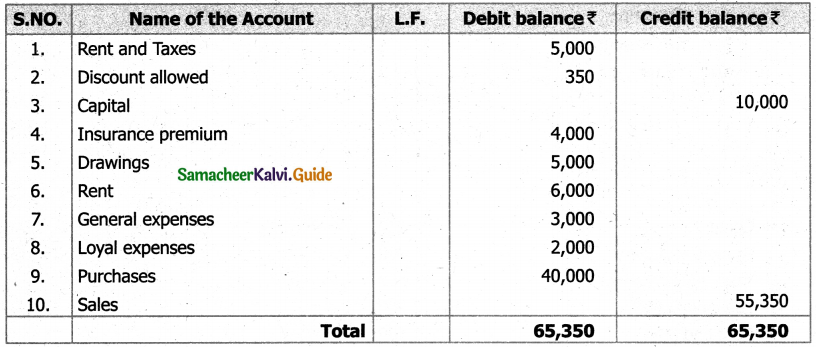Question 8.
From the following balances of Rohini, prepare the trial balance as on 31st March, 2016.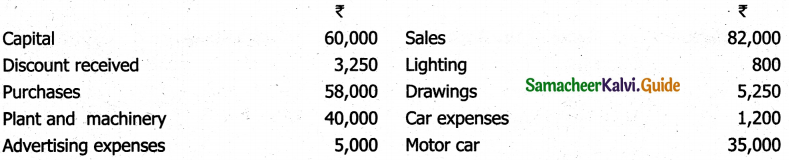Solution:
In the books of Rohini Trial Balance as on 31st March 2018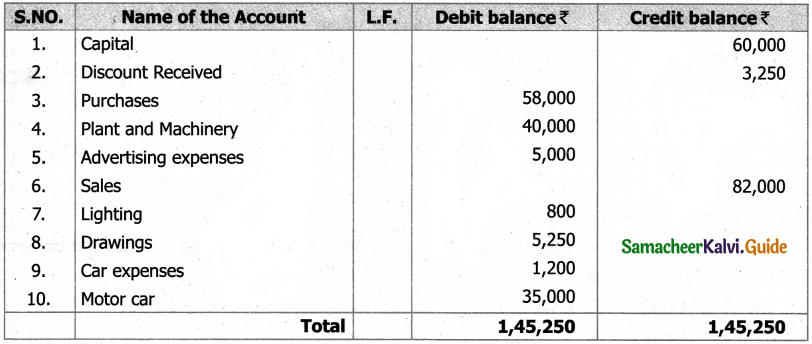Question 9.
Balan who has a car driving school gives you the following ledger balances. Prepare trial balance as on 31st December, 2016.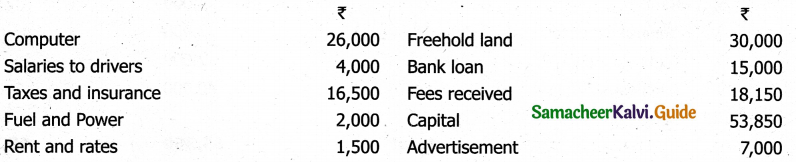Solution:
In the books of Balan Trial Balance as on 31st December 2016.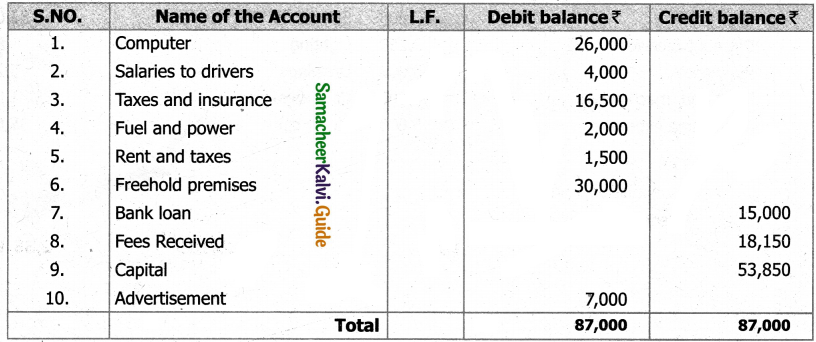Question 10.
The following balances are extracted from the books of Ravichandran on 31st December, 2016.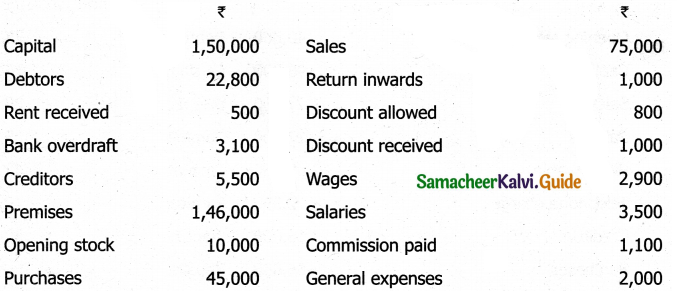Solution:
In the books of Ravichandran Trial Balance as on 31st December 2016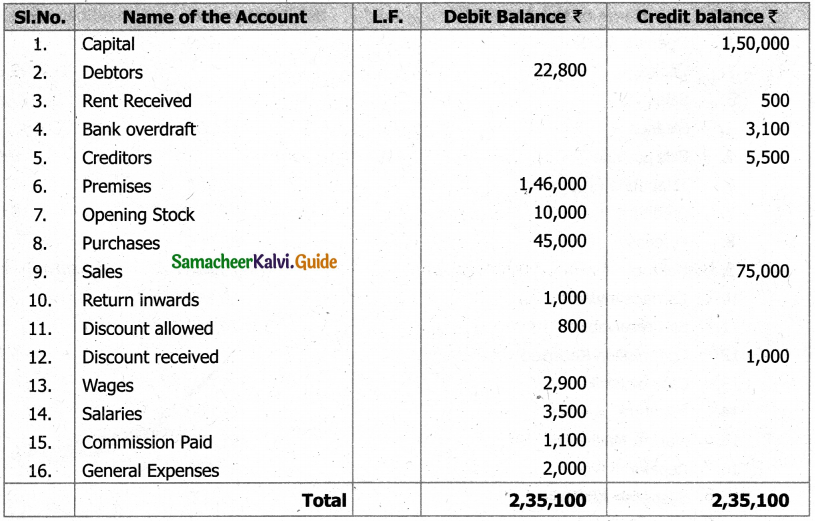Question 11.
From the following balances, prepare trial balance of Baskar as on 31st March, 2017. Transfer the difference, if any, to suspense account.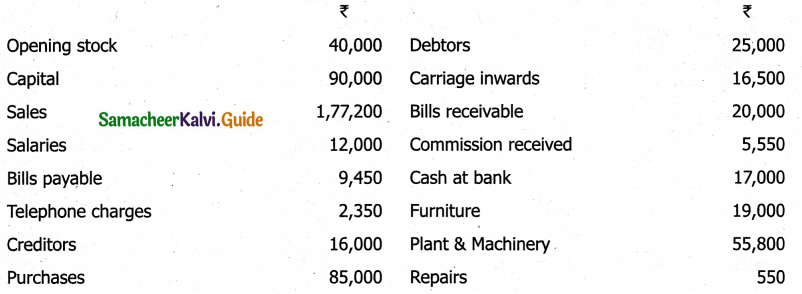Solution:
In the books of Baskar Trial Balance as on 31st March 2017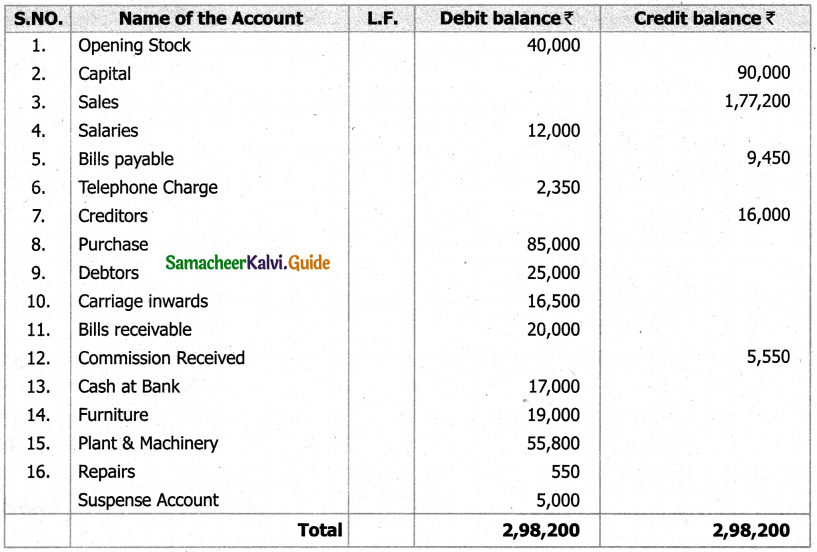Question 12.
From the following balances extracted from the books of Rajeshwari as on 31st March, 2017, prepare the trial balance.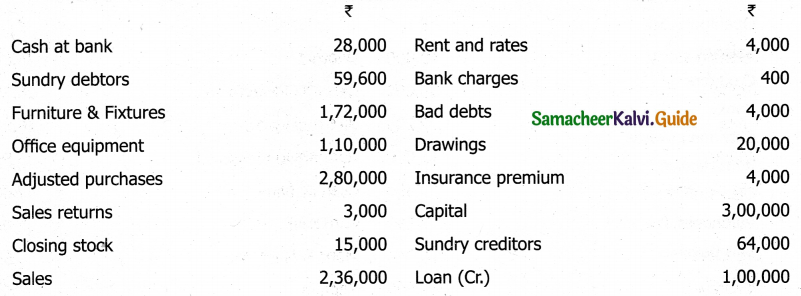Solution:
In the books of Baskar Trial Balance as on 31st March 2017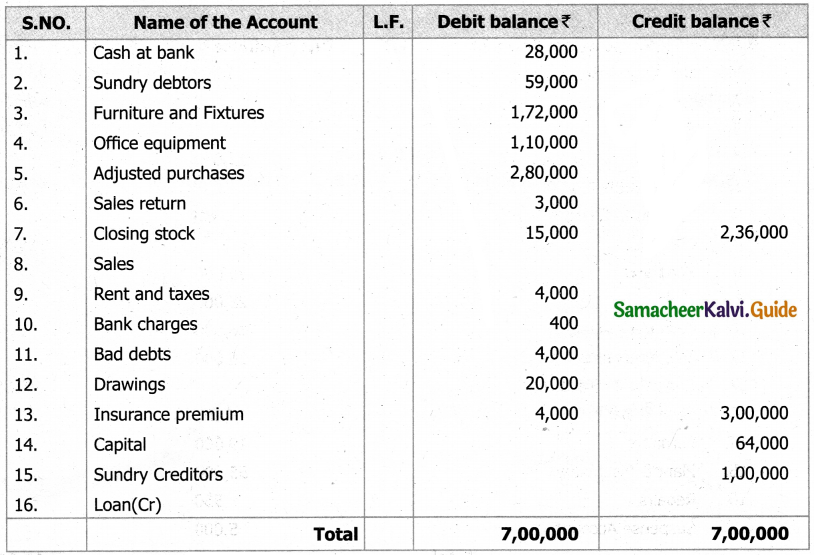Question 13.
Correct the following trial balance.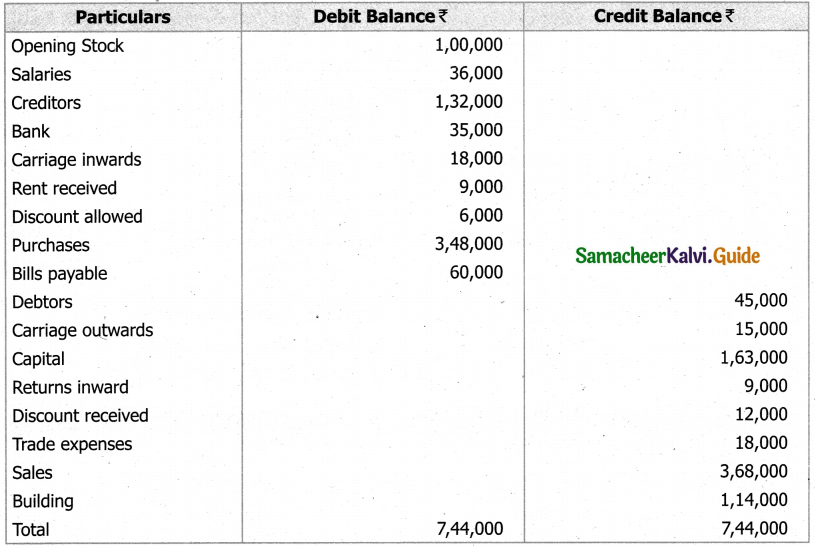Solution:
Trial Balance: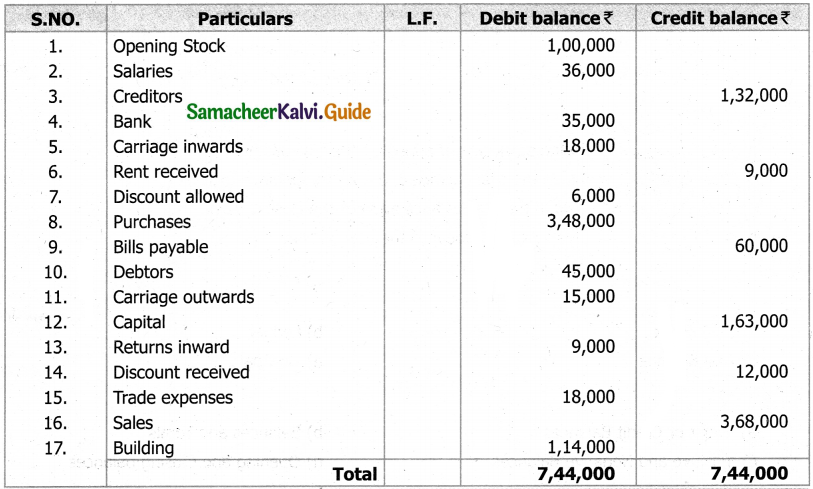Question 1.
Trial Balance shows arithmetical accuracy of ledger accounts, but it is not a _______ of accuracy.
a) Conclusive
b) Exclusive
c) Submissive
d) Inclusive
a) Conclusive

Question 2.
The statement showing balance of all the ledger accounts is known as _______.
a) Trial balance
b) Balance sheet
c) Bank reconciliation statement
d) Profit and loss account
a) Trial balance

Question 3.
Which of the following in Trial Balance is contradictory to each other?
a) Inventory and Drawings
b) Sales and Purchases returns
c) Carriage inwards and outward

Question 4.
Closing stock in the Trial Balance implies that _______.
b) It is adjusted in sales account
c) It is adjusted in the purchases account
d) None of these
c) It is adjusted in the purchases account

Question 5.
The preparation of trial balance is for _______.
a) Locating errors of commission
b) Locating errors of principle
c) Locating clerical errors
d) All of the above
d) All of the above

Question 6.
_______ Us Hie most popular method of preparing trial balance.
a) Balance Method
b) Net Asset Method
c) Average Trial Balance method
d) None of the above
a) Balance MethodQuestion 7.
When adjusted purchase is shown on the debit column of the trial balance then
a) Both opening and closing stock do not appear in the trial balance
b) Closing stock is shown in the trial balance and not the opening stock
c) Opening stock is shown in the trial balance and not the closing stock
d) Both opening and closing stock appear in the trial balance
b) Closing stock is shown in the trial balance and not the opening stock

Question 8.
A suspense account facilitates the preparation of financial statements even when the has not tallied.
a) Cash Book
b) Ledger
c) Trial Balance
d) Journal
c) Trial Balance

Question 9.
Trial Balance is a statement which shows _______ of all accounts.
a) Debit or Credit Balances
b) Balances and Totals
c) Positive and Negative Balances
d) Opening and Closing balances
a) Debit or Credit Balances

Question 10.
All the following statements are correct except _______.
a) Trial balance is a statement and not an account
b) Trial balance is prepared at the end of the year
c) Trial balance is not a concluding proof of arithmetical accuracy
d) Trial balance is tallied if a transaction posted twice in the ledger
c) Trial balance is not a concluding proof of arithmetical accuracy

Question 11.
Trial balance of a trader shows the following balances: Opening Inventory Rs.9600;Purchases less returns Rs.11850; Salaries Rs.3200;Wages Rs.750; Commission on purchases Rs.200; Carriage outwards Rs.300; Sales Rs.24900; Closing Inventory Rs.3500; Carriage on purchases Rs.1000; Gross profit will be .
a) Rs. 5,000
b) Rs. 6,500
c) Rs. 3,250
d) Rs. 3,200
a) Rs. 5,000

Question 12.
Trial Balance is a _______.
a) Real account
b) Nominal account
c) Personal account
d) Statement
d) Statement

Question 13.
Bank account will show _______ balance.
a) Debit
b) Credit
c) Debit (or) Credit
d) None
a) Debit

Question 14.
Which of the following will affect the agreement of a trial balance?
a) Complete omission of a transaction
b) Partial omission of a transaction
c) Error of principle
d) Compensating errors
b) Partial omission of a transaction

Question 15.
Trial balance is prepared to find out the _______.
a) Profit
b) loss
c) financial position
d) Arithmetical accuracy of the accounts
d) Arithmetical accuracy of the accountsQuestion 16.
Suspense account in the trial balance is entered in the
b) Profit and loss A/c
c) Balance sheet.
d) None of the above
c) Balance sheet.

Question 17.
Suspense account having credit balance will be shown on the _______.
a) Credit side of the profit and loss A/c
b) Liabilities side of the balance sheet
c) Assets side of the balance sheet
d) Debit side of the profit and loss A/c
b) Liabilities side of the balance sheet

Question 18.
Suspense account having debit balance will be shown on the _______.
a) Credit side of the profit and loss A/c
b) Liabilities side of the balance sheet
c) Assets side of the balance sheet
d) Debit side of the profit and loss A/c
c) Assets side of the balance sheet

Question 19.
While preparing the trial balance, the accountant finds that the total of the debit column is short by Rs. 1,000. This difference will be _______.
a) Debited to suspense account
b) Credited to suspense account
c) Adjusted to any of the debit balance
d) Adjusted to any of the credit balance
a) Debited to suspense account

Question 20.
The preparation of trial balance is the step in the accounting process.
a) first
b) second
c) third
d) fourth
c) third

Question 21.
When double entry system is followed, the totals of the debit and the Credit columns of the trial balance must be _______.
a) Equal
b) unequal
c) Excess
d) Short
a) Equal

Question 22.
The account which has a credit balance and is shown in the debit column of the trial balance is
a) Sundry debtors account
b) Bills receivable account
c) Purchases account
d) Capital account
d) Capital account

Question 23.
Which one the following represents correct sequence of accounting cycle _______.
a) Journal > Trial balance > Ledger > Transaction
b) Transaction > Journal > Ledger > Trial balance
c) Purchases > Journal > Ledger > Trial balance
d) None of the above
b) Transaction > Journal > Ledger > Trial balanceQuestion 24.
Which one of the following is the most popular method of preparing a trial balance.
a) Total method
b) Balance method
c) Trial and error method
d) Line method
b) Balance method

II. Very Short Answer Type Questions

Question 1.
What are the features of Trial Balance?

1. Trial balance contains the balances of all ledger accounts.
2. It is prepared on a specific date. That is why, the word, “as on…” is used at the top.
3. When double entry system is followed, the totals of the debit and the credit columns of the trial balance must be equal.
4. If there is a difference between the totals of debit column and credit column of the trial balance, it is an indication of errors being committed somewhere.
5. If both the debit column and the credit column of the trial balance have the same total, it does not mean that there is no mistake in accounting, since some errors are not disclosed by the trial balance.

Question 2.
What are the limitations of trial balance?
The following are the limitations of trial balance:

1. It is possible to prepare trial balance of an organisation, only if the double entry system is followed.
Even if some transactions are omitted, the trial balance will tally.
2. Trial balance may tally even though errors are committed in the books of account.
3. If trial balance is not prepared in a systematic way, the final accounts prepared on the basis of trial balance may not depict the actual state of affairs of the concern.
4. Agreement of trial balance is not a conclusive proof of arithmetical accuracy of entries made in the accounting records.
5. This is because there are certain errors which are not disclosed by trial balance such as complete omission of a transaction, compensating errors and error of principle.

Question 3.
State whether the balance of the following accounts should be placed in the debit or the credit column of the trial balance:
(i) Opening Stock
(ii) Bank Loan
(iv) Good will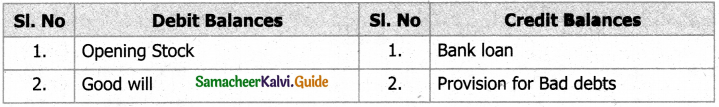Question 1.
What is a Suspense Account?
It is used as a place to hold unclassified or disputed funds and expenses. It is used to carry

Question 2.
What are the steps to be followed to prepare trial balance under Balance method?
Step 1 : Calculate the balances of all ledger accounts including the cash book.

Step 2 : Record the names of the accounts in the particulars column and the amounts of debit balances in the debit column and credit balances in the credit column.

Step 3 : Enter the page number of ledger from which the balance is taken in the Ledger Folio column.

Step 4 : Total the debit and credit columns. It must be equal. If not equal, locate the errors and make the trial balance agree.

Question 3.
State whether the balance of each of the following accounts should be placed in
The debit or the credit column of the trial balance:
(1) Sundry debtors
(2) Sundry creditors
(3) Cash in hand
(4) Bank overdraft
(5) Salary
(6) Discount allowed
(7) Plant and machinery
(8) Furniture
(9) Commission earned
(10) Reserve Fund
Solution: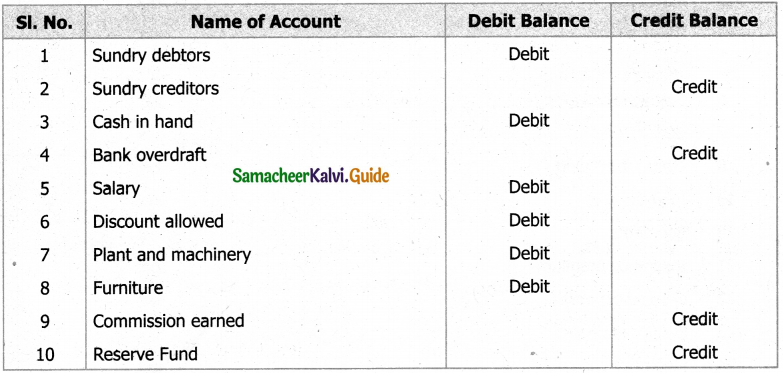Question 4.
What are the advantages of a Trial Balance?

1. It helps to ascertain the arithmetical accuracy of the book-keeping work done during the period.
2. It supplies in one place ready reference of all the balances of the ledger accounts.
3. If any error is found out by preparing a trial balance, the same can be rectified before preparing final accounts.
4. It is the basis on which final accounts are prepared.

Specimen of Trial Balance
Trial balance of Mr. X as on –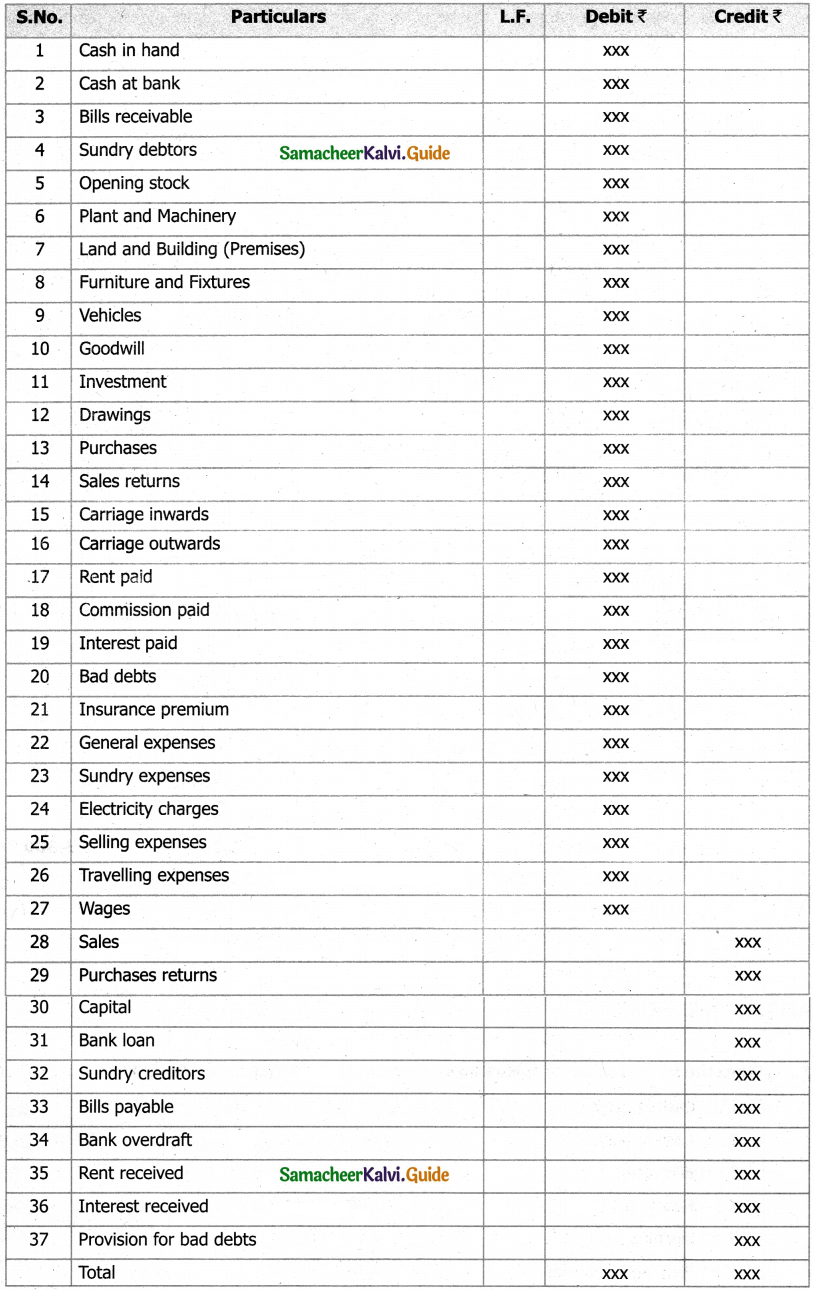Question 5.
Prepare Trial Balance with the following information’s: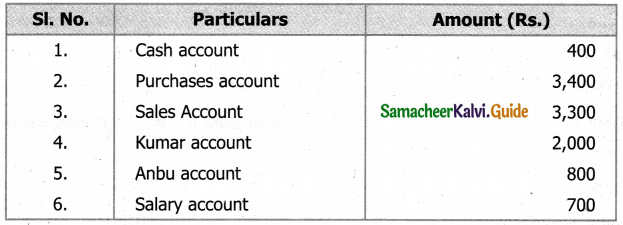Solution:
Trial Balance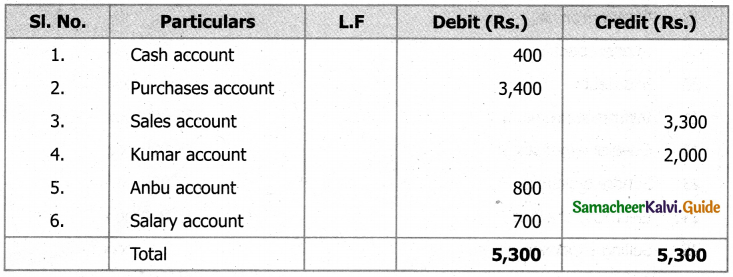IV. Exercises

Question 1.
From the following list of balances, prepare a trial balance as on 31.3.2017.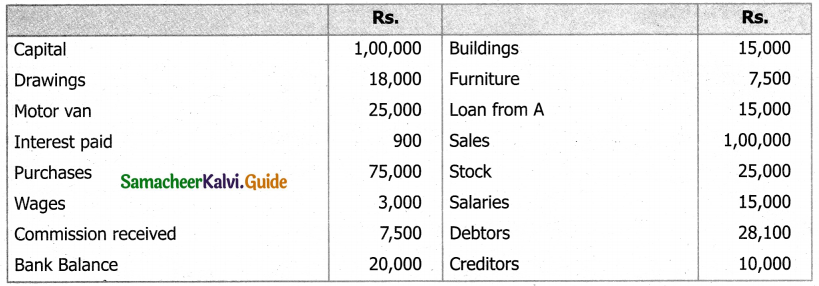Solution:
Trial Balance as on 31.3.2017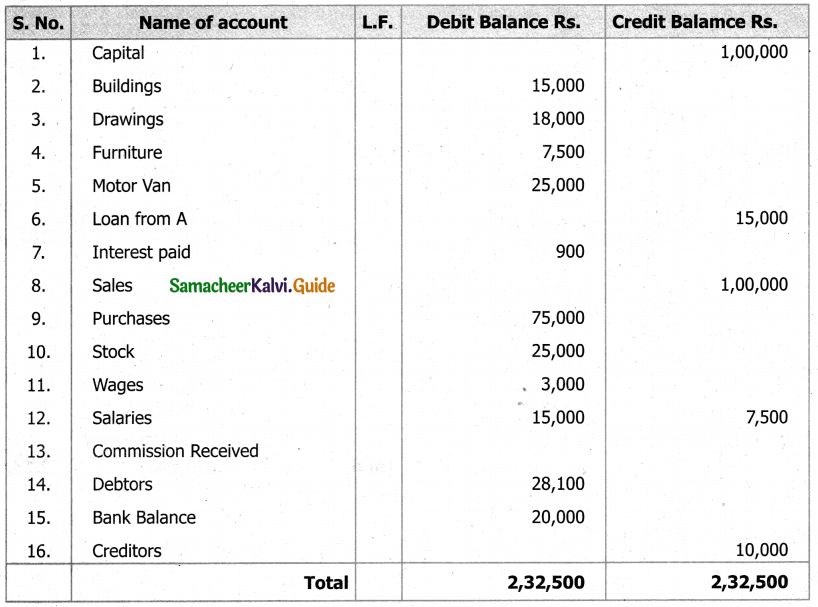Question 2.
Prepare tare trial balance from the following: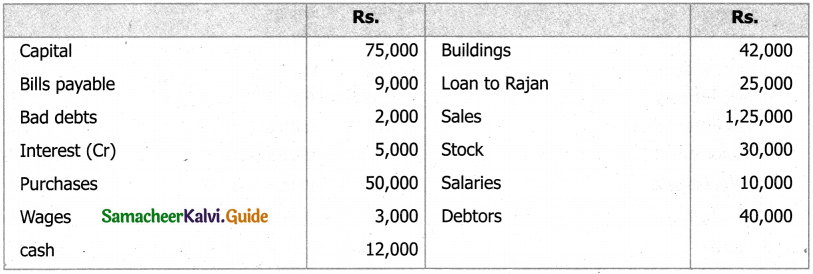Solution:
Trial Balance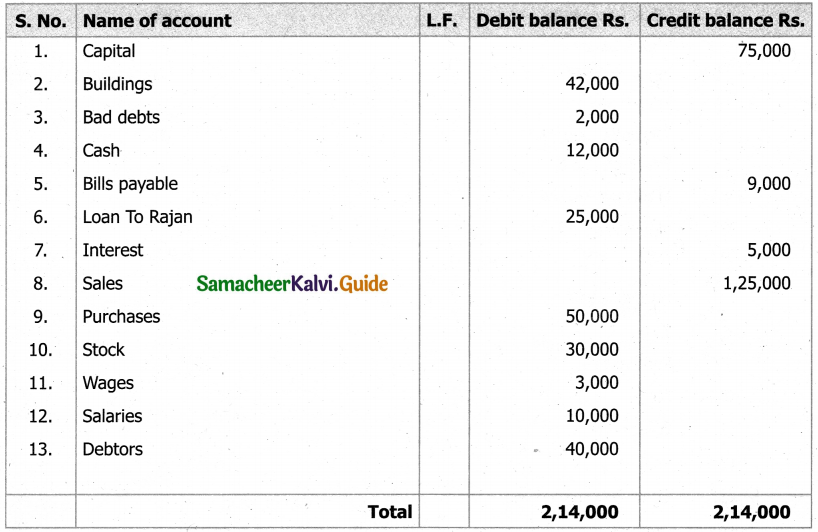Question 3.
From the following details prepare Trial Balance: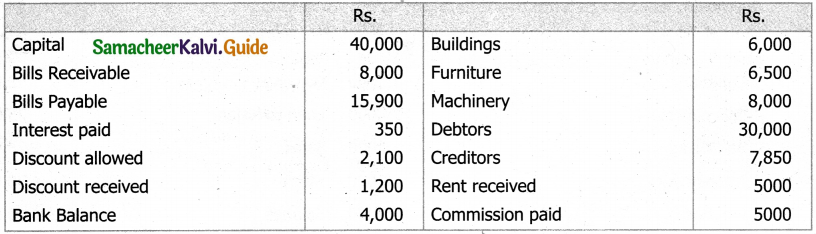Solution:
Trial Balance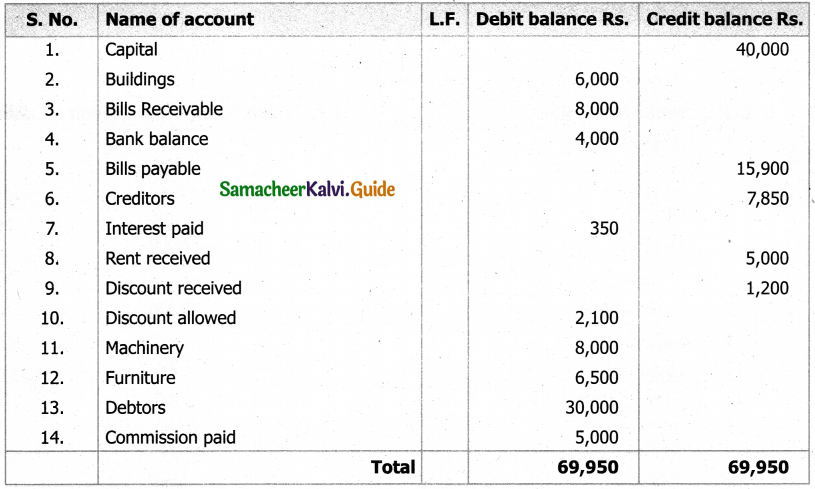Question 4.
The following are the balances extracted from the books of Mr.M as on 31st December, 2017. Prepare Trial Balance. ,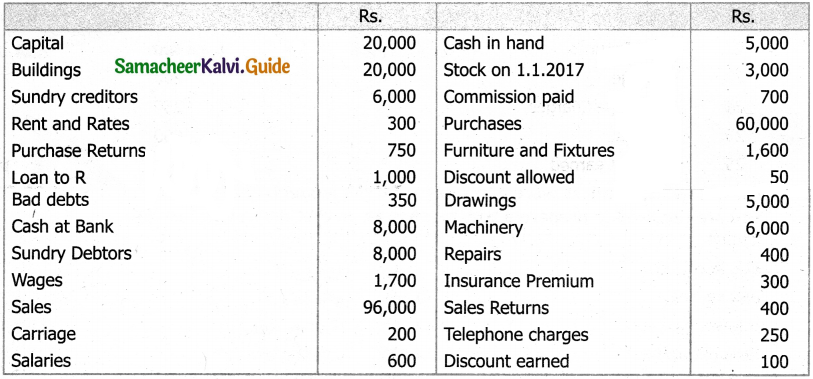Solution:
Trial Balance of Mr.M as on 31.12.2017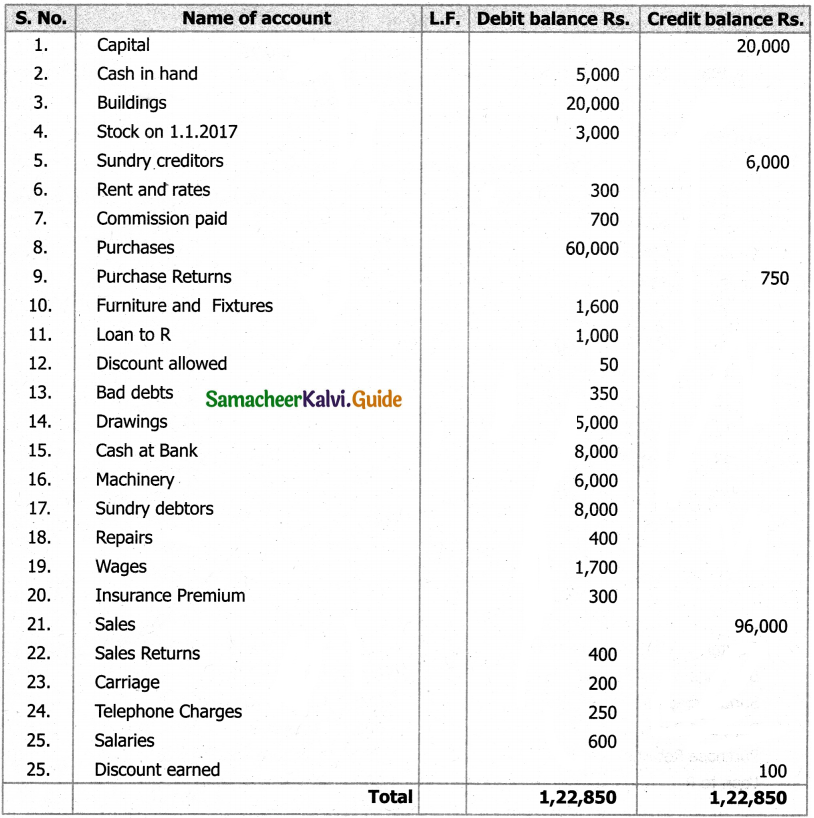Question 5.
The following balances were extracted from the ledger of Shri Prasad on 31st March 2017. You are required to prepare a trial balance as on that date in proper form.
Solution: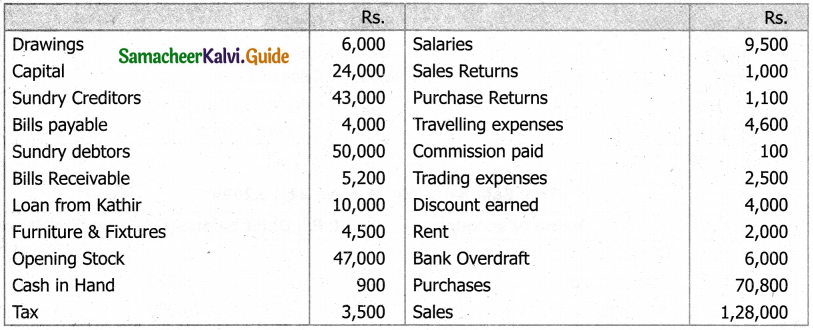Trial Balance of Shri Prasad as on 31.3.2017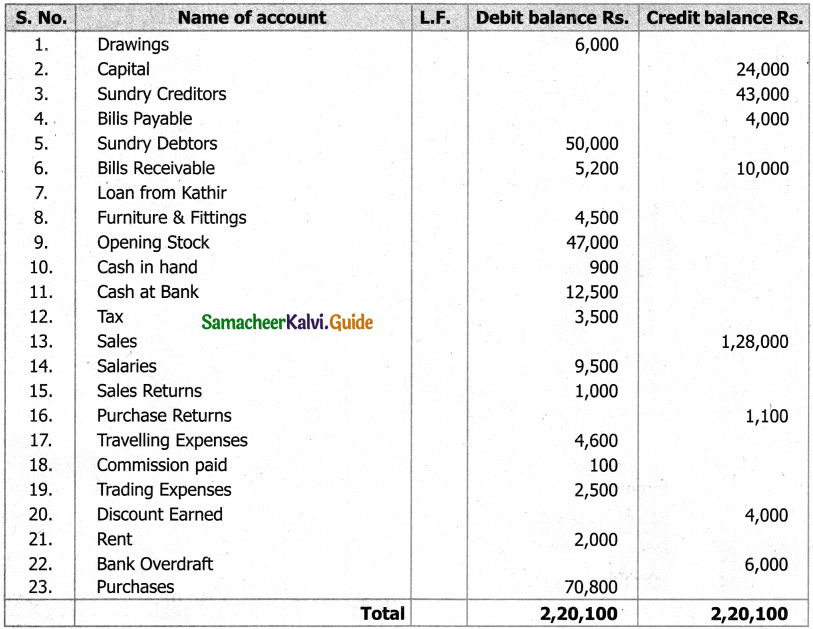Question 6.
Prepare Trail Balance.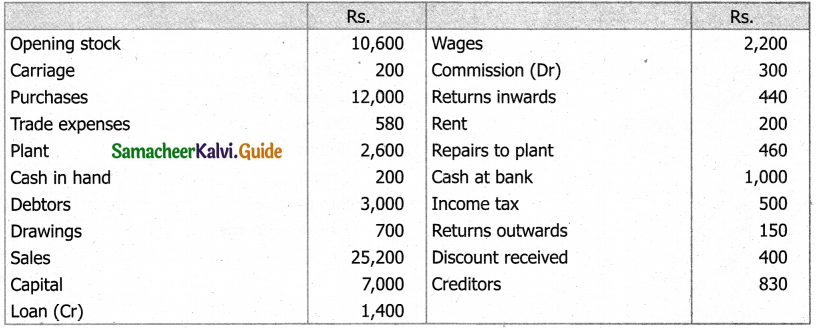Solution:
Trail Balance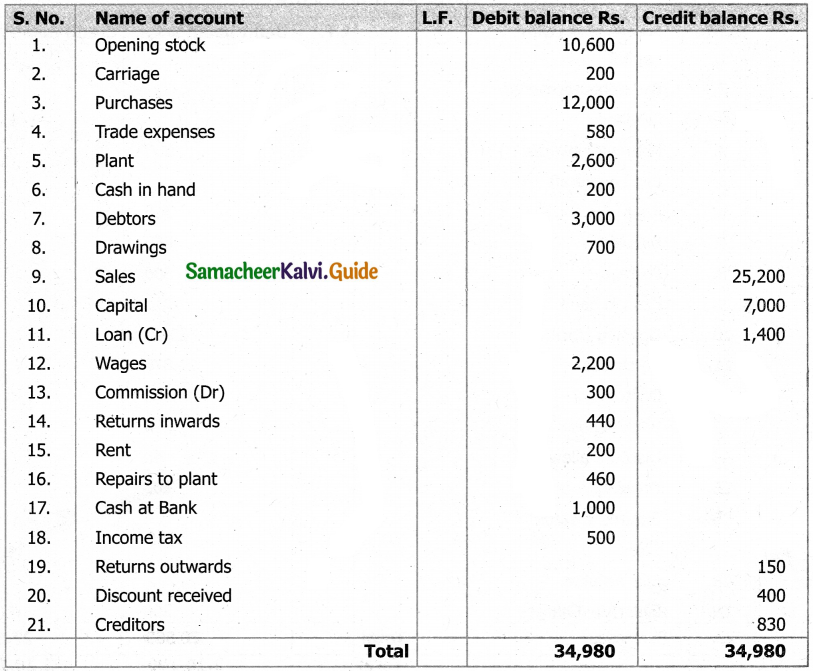Question 7.
Redraw correctly the trial balance given below: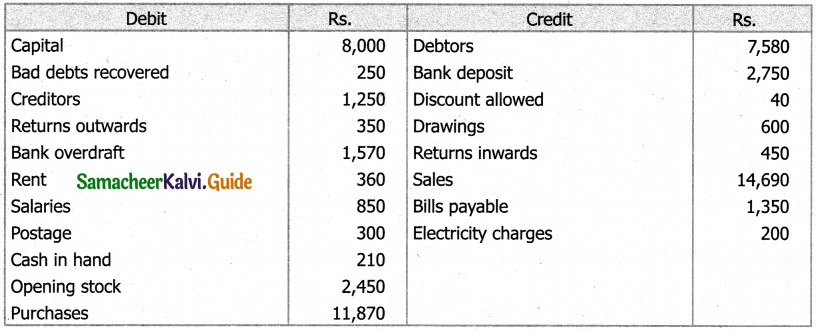Solution:
Corrected Trial Balance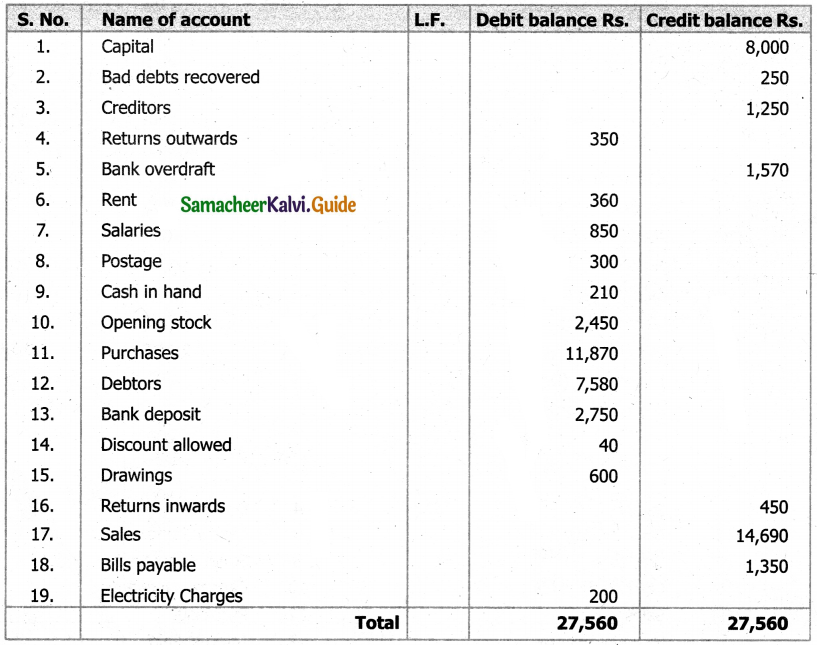Question 8.
From the following list of balances, prepare Trial Balance: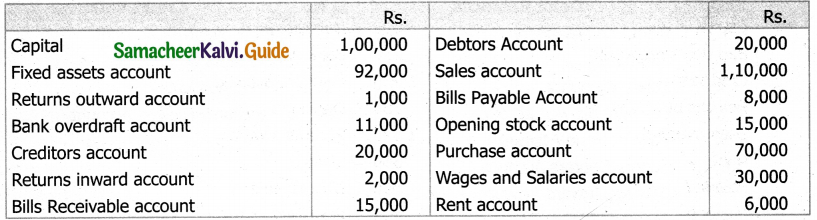Solution:
Trial Balance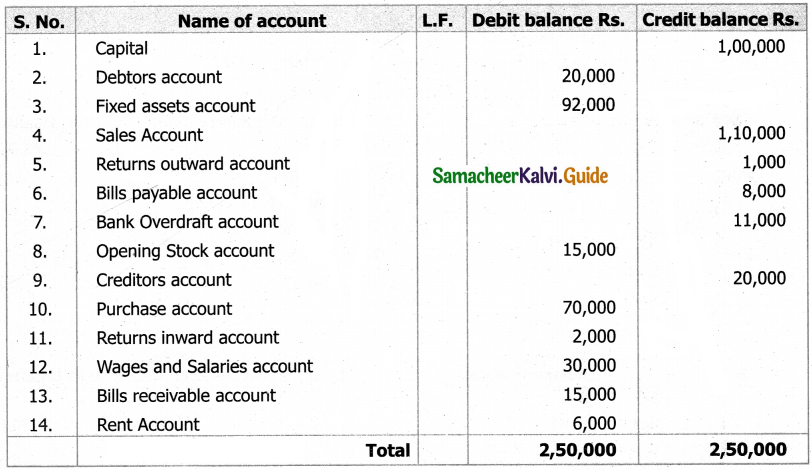Question 9.
State whether the balances of the following accounts should be placed in debit column or credit column of the trial balance.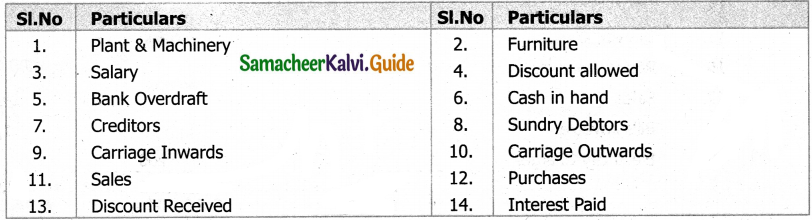Solution: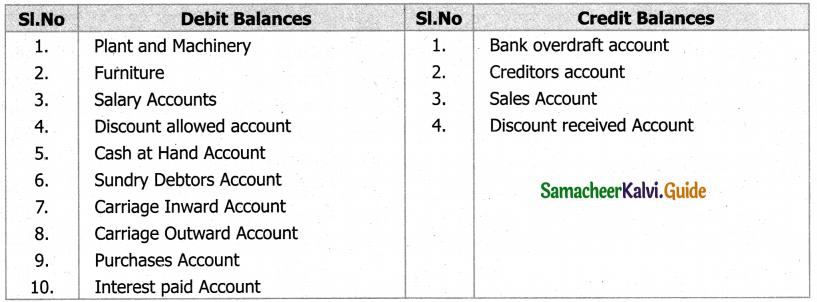Question 10.
From the under mentioned balances extracted from the books of a sole trader on 31st December. Prepare a Trial Balance as on 31st December, 2017.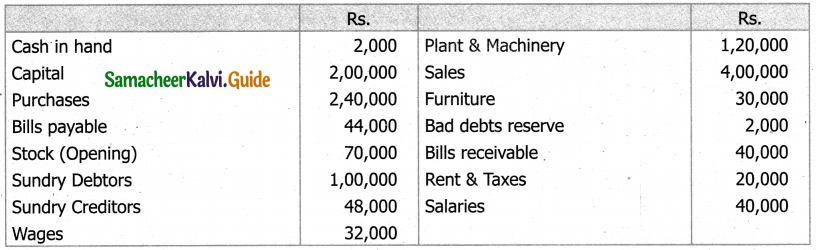Solution: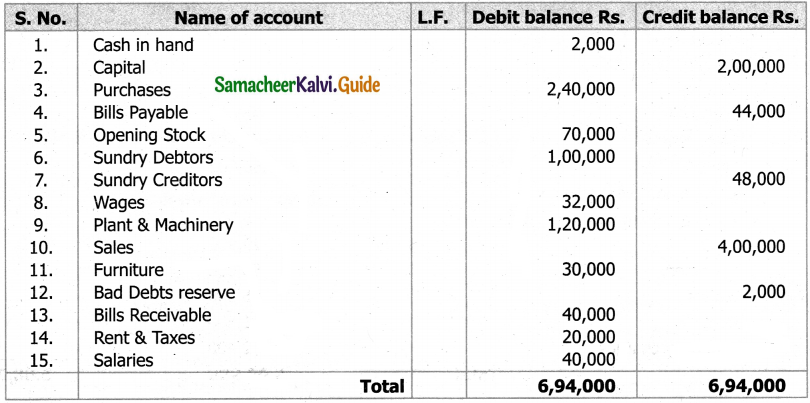Question 11.
Prepare trial balance as on 31.12.2000 From the followings balances of Mr. Kishore Kumar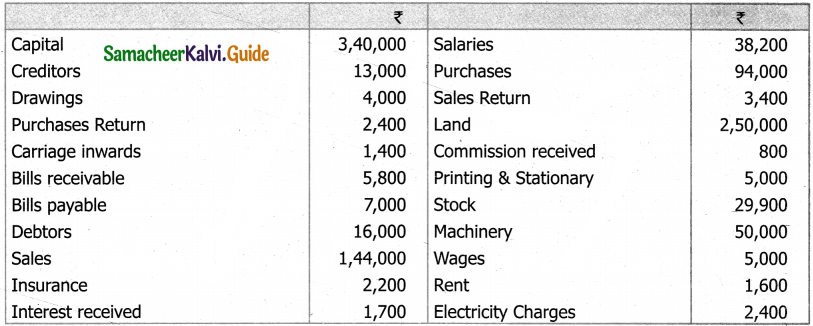Solution:
In the book of Mr. Kishore Kumar Trial balance as on 31st December 2000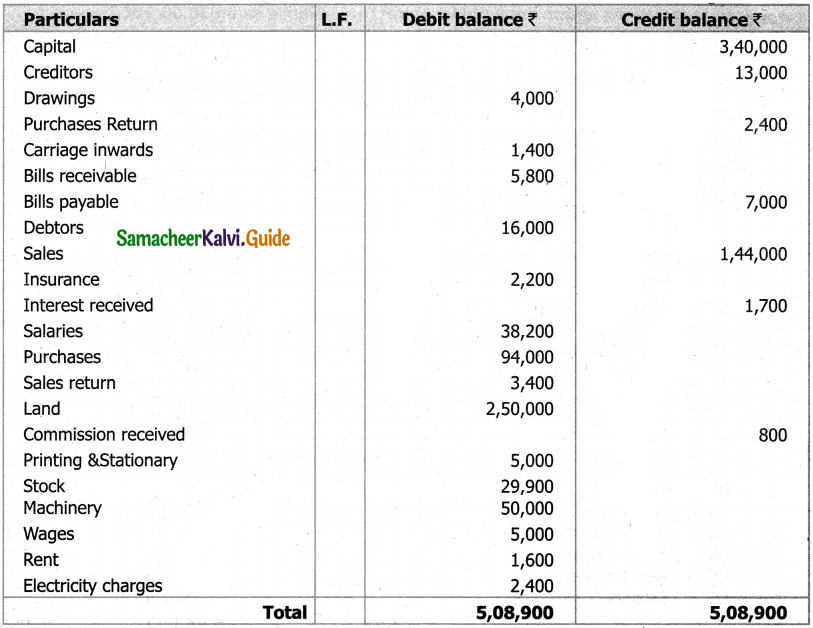Question 12.
The following balances are extracted from the books of Mr. Anbu. Prepare trial balance as on 30.6.2004.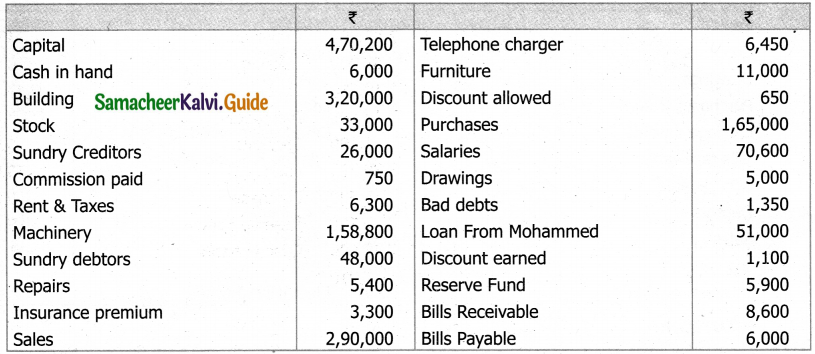Solution:
In the book of Mr. Anbu trial balance as on 30.06.2004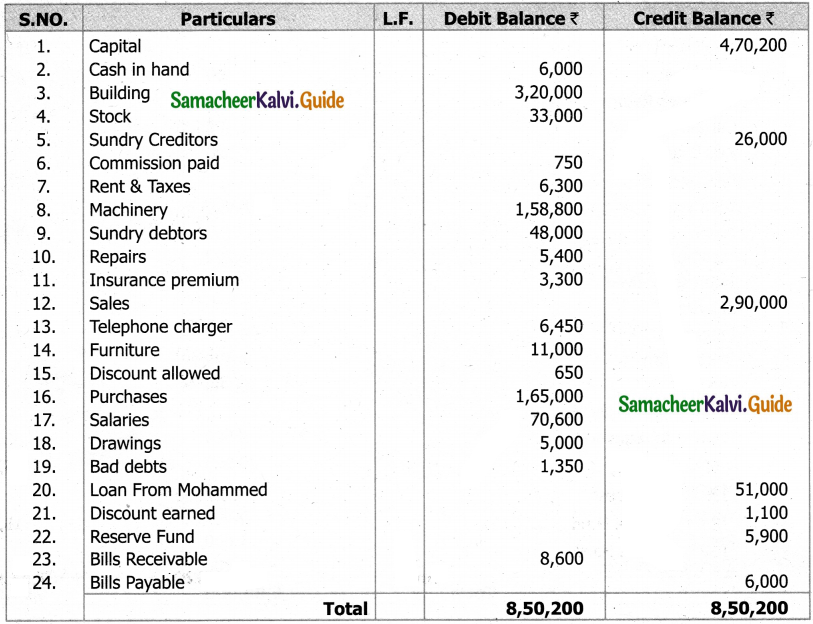Question 13.
Prepare Trial Balance as on 31.3.2004 From the books of Mr. Praveen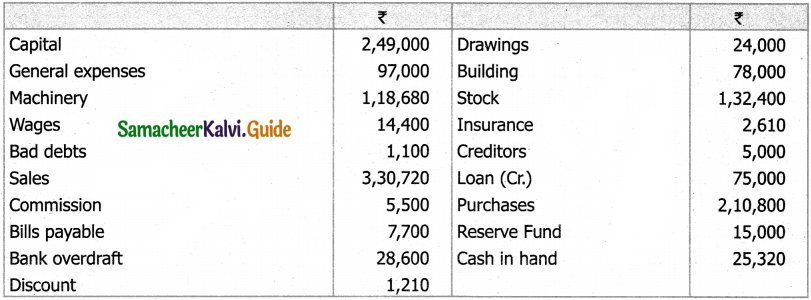Solution:
Prepare Trail Balance as 31.03.2004 from the books Mr. Praveen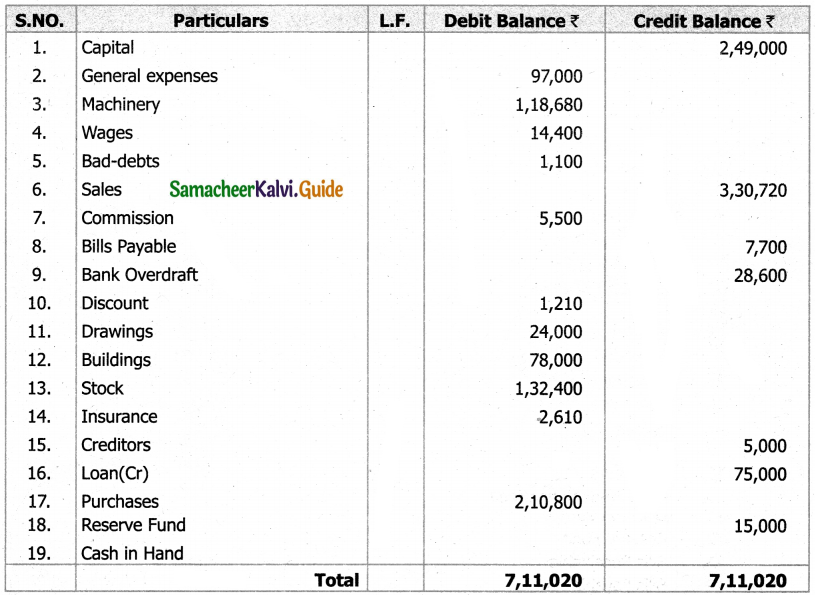Question 14.
Prepare trial balance as on 31.12.2002. From the followings balance of Mr. Ram Prasanth.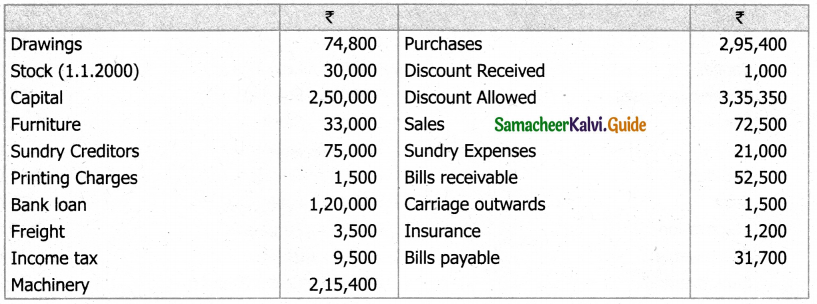Solution:
In the books of Mr. Ram Prasanth trial balance as on 31.12.2002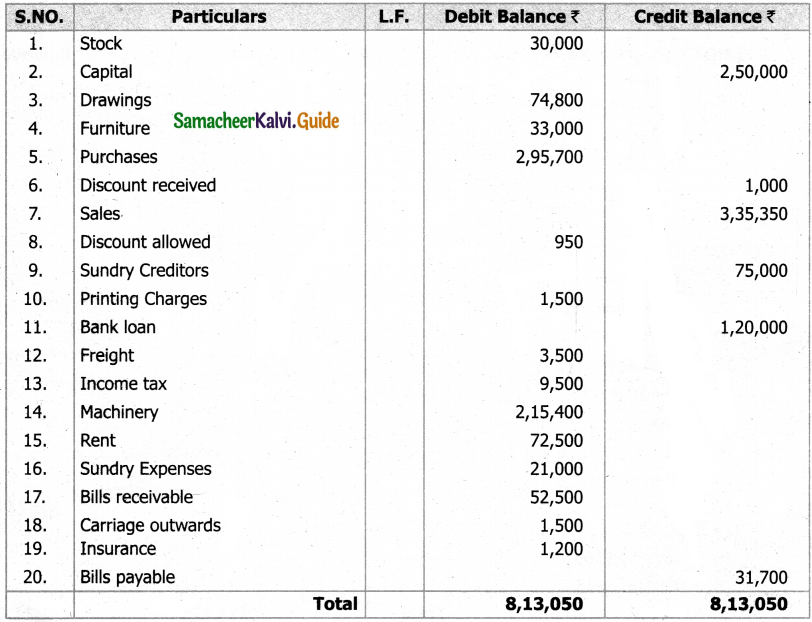Question 15.
From the extracats of Mr.Keerthivasan books of accounts prepare Trail balance as on 31.03.94.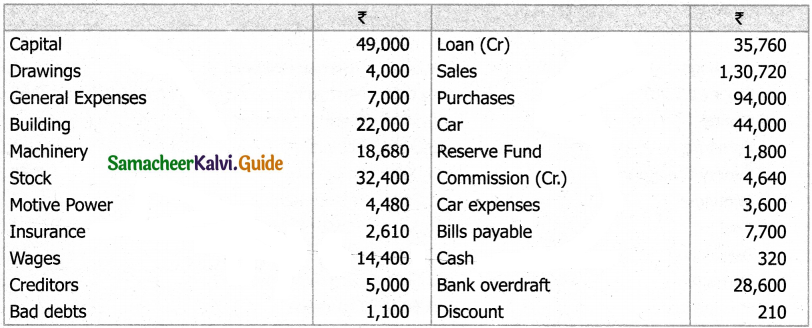In the books of Mr. Keerthivasan trial balance as on 31.03.1994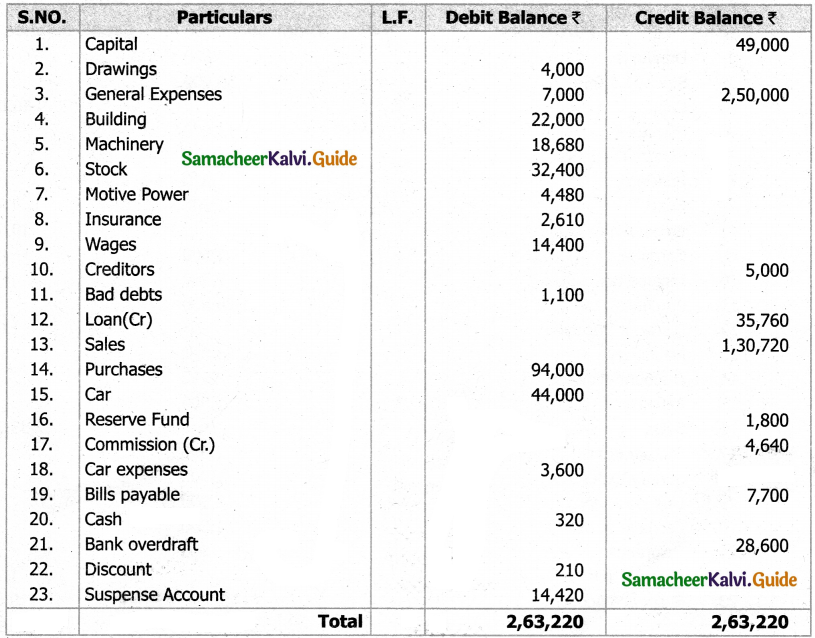Question 16.
The Following details have been extracted from Mr. Kirubakaran books of accounts as on 31.12.98.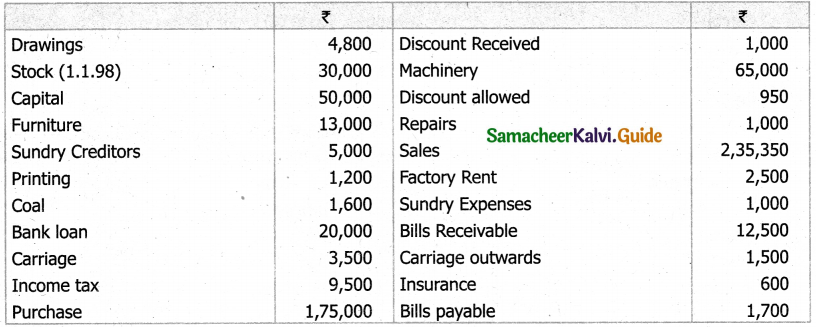Solution:
In the books of Mr. Kirubakaran trial balance as on 31.12.1998